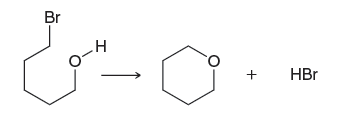Problem: Consider the following reaction:Use Table 6.1 to estimate ΔH for this reaction.

FREE Expert Solution
86% (371 ratings)
Problem Details

Consider the following reaction:

Use Table 6.1 to estimate ΔH for this reaction.What scientific concept do you need to know in order to solve this problem?

Our tutors have indicated that to solve this problem you will need to apply the Enthalpy concept. You can view video lessons to learn Enthalpy Or if you need more Enthalpy practice, you can also practice Enthalpy practice problems .

What is the difficulty of this problem?

Our tutors rated the difficulty of Consider the following reaction:Use Table 6.1 to estimate ΔH... as medium difficulty.

What professor is this problem relevant for?

Based on our data, we think this problem is relevant for Professor Teske's class at WMU.

What textbook is this problem found in?

Our data indicates that this problem or a close variation was asked in . You can also practice practice problems .